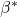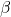# Properties of Good Estimator

Small Sample properties

Unbiased Estimator : Biased means the difference of true value of parameter and value of estimator. When the difference becomes zero then it is called unbiased estimator. i.e.,Best Estimator : An estimator is called best when value of its variance is smaller than variance is best. i.e. Whereis another estimator.

Efficient Estimator : An estimator is called efficient when it satisfies following conditionsis Unbiased i.eThe estimator is best i.eLinear Estimator : An estimator is called linear when its sample observations are linear function. For Examplethen. Where k are constants.

BLUE : An estimator is BLUE when it has three properties :

1. Estimator is Linear.
2. Estimator is Unbiased.
3. Estimator is Best

So an estimator is called BLUE when it includes best linear and unbiased property.

MSE Estimator : The meaning of MSE is minimum mean square error estimator. It is the combinations of unbiasedness and best properties. An estimator is called MSE when its mean square error is minimum.

The formula for calculating MSE is MSE() = var+Sufficient Estimator : An estimator is called sufficient when it includes all above mentioned properties, but it is very difficult to find the example of sufficient estimator. Only arithmetic mean is considered as sufficient estimator.

Large Sample properties

A sample is called large when n tends to infinity. This property is called asymptotic property. The large sample properties are :

Asymptotic Unbiasedness : In a large sample if estimated value of parameter equal to its true value then it is called asymptotic unbiased. i.e.,whenConsistency : An estimators called consistent when it fulfils  following two conditions

1.must be Asymptotic Unbiased.
2. The variance ofmust approach to Zero as n tends to infinity.

Asymptotic Efficiency : An estimatoris called asymptotic efficient when it fulfils following two conditions :must be Consistent., whereandare consistent estimators.